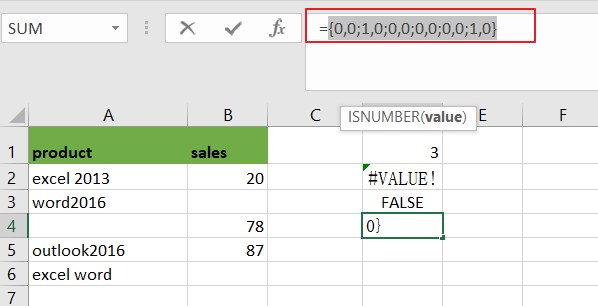# How to Count Cells that Contain X or Y in Excel

This post will guide you how to count the number of cells that contain X or Y in a given range cells using a formula in Excel 2013/2016.You can easily to count cells equal to a specific value or text string through COUNTIF function. But if there is an easy way to count cells contain either one value or another value in a selected range of cells in Excel.

In the previous post, we talked that how to count cells that equal to either x or y in a given range with two COUNTIF functions. And it will double count cells that contain both X and Y. So it may be not your expected result, and you may only want to add it once while cells that contain both X or Y. the below steps will teach you how to count cells that contain either X or Y(do not double count).

## Count Cells Contain X or Y

Assuming that you want to count cells that contain value “excel”or “word” defined in a selected range(A1:B6).In this case, you can use the SUMPRODUCT function combining with ISNUMBER function and FIND function to count cells which are contain either  X or Y.

Enter the following formula in a blank cell, and press Enter key:

=SUMPRODUCT(–((ISNUMBER(FIND(“excel”,A1:B6)) +  ISNUMBER(FIND(“word”,A1:B6)))>0))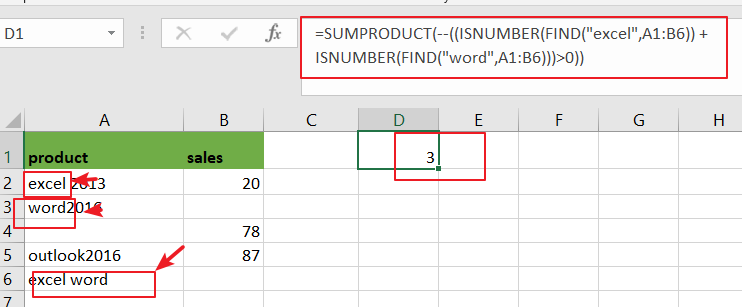Note: A2:A6 is the data range that you want to use. And both“excel” and “word” are the text values that you want to count in range A2:A6. You need to change them as you need.

NOW LET’S SEE HOW THIS FORMULA WORKS:

=FIND(“excel”,A1:B6)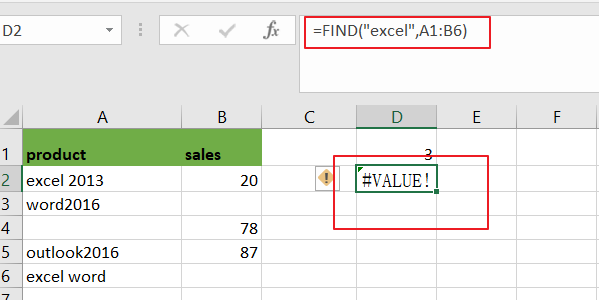The FIND function can be used to find the position of text “excel” or “word” in range A1:B6, and it returns a array result contain `1` or `#VALUE` error. When the text “excel” or “word” is not found, returns a `#VALUE` error. The returned results for the above formula is like below:

={#VALUE!,#VALUE!;1,#VALUE!;#VALUE!,#VALUE!;#VALUE!,#VALUE!;#VALUE!,#VALUE!;1,#VALUE!}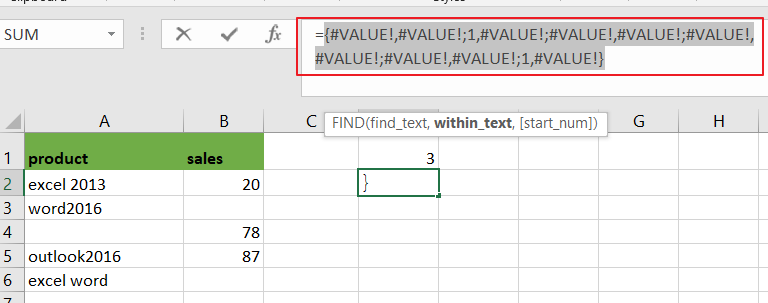You can see that there are two “`1`” value, it means that there are two cells that contain “excel”text string.

=ISNUMBER(FIND(“excel”,A1:B6))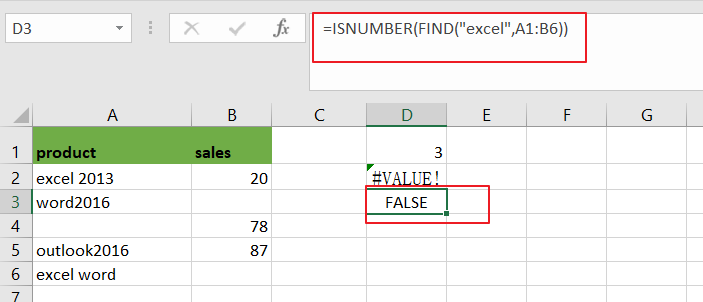Then you can use the ISNUMBER function to check the above array result, it will convert all numeric value as True, and convert other values to False.

={FALSE,FALSE;TRUE,FALSE;FALSE,FALSE;FALSE,FALSE;FALSE,FALSE;TRUE,FALSE}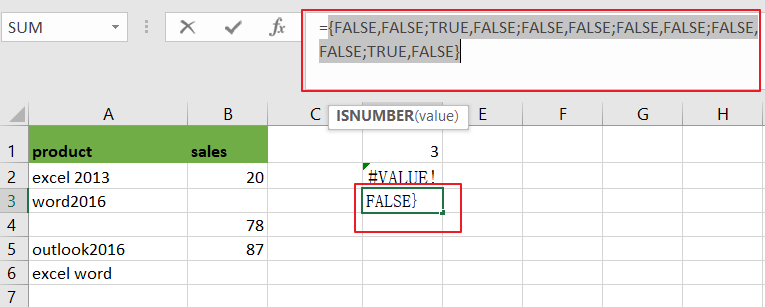You still need to convert the above logic values to number “`1`” or “`0`” with double negative character like below:

=–ISNUMBER(FIND(“excel”,A1:B6))

={0,0;1,0;0,0;0,0;0,0;1,0}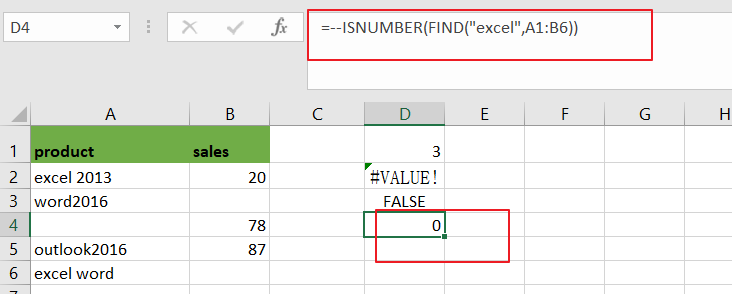Note: You can press “Fn” + “F9” to display the array result for the above array formula.

### Related Functions

• Excel SUMPRODUCT function
The Excel SUMPRODUCT function multiplies corresponding components in the given one or more arrays or ranges, and returns the sum of those products. The syntax of the SUMPRODUCT function is as below:= SUMPRODUCT (array1,[array2],…)…
• Excel COUNTIF function
The Excel COUNTIF function will count the number of cells in a range that meet a given criteria. This function can be used to count the different kinds of cells with number, date, text values, blank, non-blanks, or containing specific characters.etc.= COUNTIF (range, criteria)…
• Excel Find function
The Excel FIND function returns the position of the first text string (substring) from the first character of the second text string.The FIND function is a build-in function in Microsoft Excel and it is categorized as a Text Function.The syntax of the FIND function is as below:= FIND  (find_text, within_text,[start_num])…
• Excel ISNUMBER function
The Excel ISNUMBER function returns TRUE if the value in a cell is a numeric value, otherwise it will return FALSE.The syntax of the ISNUMBER function is as below:= ISNUMBER (value)…